Tamilnadu State Board New Syllabus Samacheer Kalvi 11th Physics Guide Pdf Chapter 10 Oscillations Text Book Back Questions and Answers, Notes.

## Tamilnadu Samacheer Kalvi 11th Physics Solutions Chapter 10 Oscillations

### 11th Physics Guide Oscillations Book Back Questions and AnswersI. Multiple choice questions:

Question 1.
In a simple harmonic oscillation, the acceptation against displacement for one complete oscillation will be: (Model NSEP 2000 – 01)
(a) an ellipse
(b) a circle
(c) a parabola
(d) a straight line
(d) a straight line

Hint:
The sketch between cause (magnitude of acceleration) and effect (magnitude of displacement) is a straight line.

Question 2.
A particle executing SHM crosses points A and B with the same velocity. Having taken 3 s in passing from A to B, it returns to B after another 3 s. The time period is:
(a) 15 s
(b) 6 s
(c) 12 s
(d) 9 s
(c) 12 s

Hint: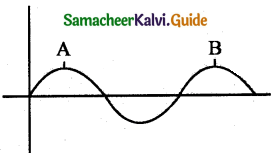The time period is the time taken by a particle to return to B.

Question 3.
The length of a second’s pendulum on the surface of the Earth is 0.9 m. The length of the same pendulum on the surface of planet X such that the acceleration of the planet X is n times greater than the Earth is:
(a) 0.9 n
(b) $$\frac { 0.9 }{ n }$$m
(c) 0.9 n²m
(d) $$\frac{0.9}{n^{2}}$$
(a) 0.9 n

Hint:Question 4.
A simple pendulum is suspended from the roof of a school bus which moves in a horizontal direction with an acceleration a, then the time period is:
(a) T ∝ $$\frac{1}{g^{2}+a^{2}}$$
(b) T ∝ $$\frac{1}{\sqrt{g^{2}+a^{2}}}$$
(c) T ∝ $$\sqrt{g^{2}+a^{2}}$$
(d) T ∝ (g² + a²)
(b) T ∝ $$\frac{1}{\sqrt{g^{2}+a^{2}}}$$

Hint: T = 2π$$\sqrt{\frac{l}{g}}$$
When a bus is moving
g’ = $$\sqrt{g^{2}+a^{2}}$$
∴ T ∝ $$\frac{1}{\sqrt{g^{2}+a^{2}}}$$Question 5.
Two bodies A and B whose masses are in the rati0 1:2 are suspended from two separate massless springs of force constants kA and kB respectively. If the two bodies oscillate vertically such that their maximum velocities are in the ratio 1:2, the ratio of the amplitude A to that of B is:
(a) $$\sqrt{\frac{k_{\mathrm{B}}}{2 k_{\mathrm{A}}}}$$
(b) $$\sqrt{\frac{k_{\mathrm{B}}}{8 k_{\mathrm{A}}}}$$
(c) $$\sqrt{\frac{2k_{\mathrm{B}}}{ k_{\mathrm{A}}}}$$
(d) $$\sqrt{\frac{8k_{\mathrm{B}}}{ k_{\mathrm{A}}}}$$
(b) $$\sqrt{\frac{k_{\mathrm{B}}}{8 k_{\mathrm{A}}}}$$

Hint: vA : vB
Amplitude of A : Amplitude of B = $$\sqrt{\mathrm{K}_{\mathrm{B}}}: \sqrt{8 \mathrm{~K}_{\mathrm{A}}}$$

Question 6.
A spring is connected to a mass m Suspended from it and its time period for vertical oscillation is T. The spring is now cut into two equal halves and the same mass is suspended from one of the halves. The period of vertical oscillation is:
(a) T’ = $$\sqrt{2}$$T
(b) T’ = $$\frac{\mathrm{T}}{\sqrt{2}}$$
(c) T’ = $$\sqrt{2T}$$
(d) T’ = $$\sqrt{\frac{\mathrm{T}}{2}}$$
(b) T’ = $$\frac{\mathrm{T}}{\sqrt{2}}$$

Hint:
T = 2π$$\sqrt{\frac{\mathrm{m}}{k}}$$
When the spring is cut into two equal halves, then the force constant of each part is 2k.
When the mass is suspended from one of the halves.
Time period T’ = 2π$$\sqrt{\frac{\mathrm{m}}{2k}}$$
= $$\sqrt{\frac{\mathrm{T}}{2}}$$

Question 7.
The time period for small vertical oscillations of block of mass m when the masses of the pulleys are negligible and spring constant k1 and k2 is: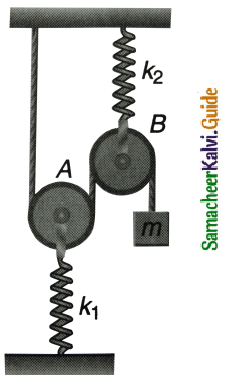(a) T = 4π$$\sqrt{m\left(\frac{1}{k_{1}}+\frac{1}{k_{2}}\right)}$$
(b) T = 2π$$\sqrt{m\left(\frac{1}{k_{1}}+\frac{1}{k_{2}}\right)}$$
(c) T = 4π$$\sqrt{m\left(k_{1}+k_{2}\right)}$$
(d) T = 2π$$\sqrt{m\left(k_{1}+k_{2}\right)}$$
(a) T = 4π$$\sqrt{m\left(\frac{1}{k_{1}}+\frac{1}{k_{2}}\right)}$$

Hint:
T = 2π$$\frac { m }{ k }$$
The given arrangement is similar to the combination of springs in series.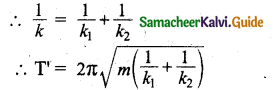Question 8.
A simple pendulum has a time period T1. When its point of suspension is moved vertically upwards according as y = kt², where y is vertical distance covered and k = 1 ms-2, its time period becomes T2. Then, $$\frac{\mathrm{T}_{1}^{2}}{\mathrm{~T}_{2}^{2}}$$ is (g = 10 ms-2
(a) $$\frac { 5 }{ 6 }$$
(b) $$\frac { 11 }{ 10 }$$
(c) $$\frac { 6 }{ 5 }$$
(d) $$\frac { 5 }{ 4 }$$
(c) $$\frac { 6 }{ 5 }$$

Hint:Question 9.
An ideal spring of spring constant k, is suspended from the ceiling of a room and a block of mass m is fastened to its lower end. If the block is released when the spring is un-stretched, then the maximum extension in the spring is:
(a) 4$$\frac { mg }{ k }$$
(b) $$\frac { mg }{ k }$$
(c) 2$$\frac { mg }{ k }$$
(d) $$\frac { mg }{ 2k }$$
(c) 2$$\frac { mg }{ k }$$

Hint: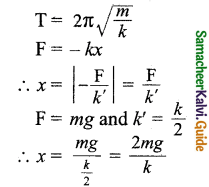Question 10.
A pendulum is hung in a very high building oscillates to and fro motion freely like a simple harmonic oscillator. If the acceleration of the bob is 16 ms-2 at a distance of 4 m from the mean position, then the time period is: (NEET 2018 model)
(a) 2s
(b) 1s
(c) 2 πs
(d) πs
(d) πs

Hint:
a = 16 m/s²; y = 4
a = – $$\frac { g }{ l }$$x = – ω²x
16 = $$\left|1-\omega^{2} \times 4\right|$$
∴ ω² = $$\frac { 16 }{ 4 }$$ = 4
ω = 2
Time period T = $$\frac { 2π }{ ω }$$ = πsQuestion 11.
A hollow sphere is filled with water. It is hung by a long thread. As the water flows out of a hole at the bottom, the period of oscillation will:
(a) first increase and then decrease
(b) first decrease and then increase
(c) increase continuously
(d) decrease continuously
(a) first increase and then decrease

Hint:
As the water flows out of the sphere, the time period first increases and then decreases. Initially, when the sphere is completely filled with water its centre of gravity (C.G) lies at its centre. As water flows out, the C.G begins to shift below the centre of the sphere.

The effective length of the pendulum increases and hence time period increases. When the sphere becomes more than half empty, its C.G begins to rise up. The effective length of the pendulum decreases and T decreases.

Question 12.
The damping force on an oscillator is directly proportional to the velocity. The units of the constant of proportionality are: (AIPMT 2012)
(a) kg m s-1
(b) kg m s-2
(c) kg s-1
(d) kg s
(c) kg s-1

Hint:
Fd ∝ v
Fd = – bv
Fd = kv
∴ k = $$\frac{\mathrm{F}_{d}}{\nu}$$
Units of k = $$\frac{\mathrm{kgms}^{-2}}{\mathrm{~m} / \mathrm{s}}$$
= kg-1
Units of proportionality constant = kgs-1

Question 13.
When a damped harmonic oscillator completes 100 oscillations, its amplitude is reduced to$$\frac { 1 }{ 3 }$$ of its initial value. What will be its amplitude when it completes 200 oscillations?
(a) $$\frac { 1 }{ 5 }$$
(b) $$\frac { 2 }{ 3 }$$
(c) $$\frac { 1 }{ 6 }$$
(d) $$\frac { 1 }{ 9 }$$
(b) $$\frac { 2 }{ 3 }$$

Hint: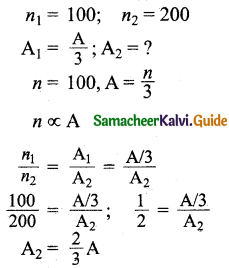Question 14.
Which of the following differential equations represents a damped harmonic oscillator?
(a) $$\frac{d^{2} y}{d t^{2}}$$ + y = 0
(b) $$\frac{d^{2} y}{d t^{2}}$$ + γ $$\frac { dy }{ dt }$$ + y = 0
(c) $$\frac{d^{2} y}{d t^{2}}$$ + k²y = 0
(d) $$\frac { dy }{ dt }$$ + y = 0
(b) $$\frac{d^{2} y}{d t^{2}}$$ + γ $$\frac { dy }{ dt }$$ + y = 0

Hint:
For a damped oscillator F ∝ v
Total restoring force F = – ky – bv
b – damping constant; y – displacement
If F = – ky – by
then m$$\frac{d^{2} y}{d t^{2}}$$ = – ky – b$$\frac { dy }{ dt }$$
m$$\frac{d^{2} y}{d t^{2}}$$ + ky + b$$\frac { dy }{ dt }$$ = 0
÷ m we get
$$\frac{d^{2} y}{d t^{2}}$$ + $$\frac { b }{ m }$$$$\frac { dy }{ dt }$$ + $$\frac { k }{ m }$$y = 0
If k = m and $$\frac { b }{ m }$$ = r
then the equation becomes
$$\frac{d^{2} y}{d t^{2}}$$ + r$$\frac { dy }{ dt }$$ + y = 0Question 15.
If the inertial mass and gravitational mass of the simple pendulum of length l are not equal, then the time period of the simple pendulum is:
(a) T = 2π$$\sqrt{\frac{m_{i} l}{m_{g} g}}$$
(b) T = 2π$$\sqrt{\frac{m_{g} l}{m_{i} g}}$$
(c) T = 2π$$\frac{m_{g}}{m_{i}} \sqrt{\frac{l}{g}}$$
(d) T = 2π$$\frac{m_{i}}{m_{g}} \sqrt{\frac{l}{g}}$$
(a) T = 2π$$\sqrt{\frac{m_{i} l}{m_{g} g}}$$

Hint: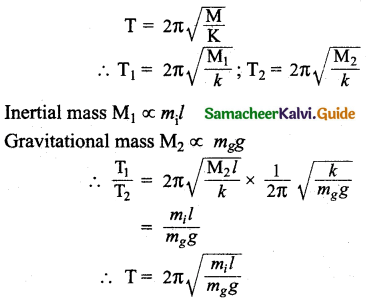Question 1.
What is meant by periodic and non-periodic motion? Give any two examples, for each motion.
Periodic motion: Any motion which repeats itself in a fixed time interval is known as periodic motion. Examples: Hands in a pendulum clock, the swing of a cradle.
Non-Periodic motion: Any motion which does not repeat itself after a regular interval of time is known as non-periodic motion. Example: Occurrence of Earthquake, the eruption of a volcano.

Question 2.
What is meant by the force constant of a spring?
Force constant is defined as force per unit length.

Question 3.
Define the time period of simple harmonic motion.
The time period is defined as the time taken by a particle to complete one oscillation. It is usually denoted by T.

Question 4.
Define frequency of simple harmonic motion.
The number of oscillations produced by the particle per second is called frequency. It is denoted by f. SI unit for frequency is s-1 or hertz (Hz).
Mathematically, frequency is related to time period by f = $$\frac{1}{\mathrm{T}}$$Question 5.
What is an epoch?
Initial phases of a particle is an epoch. At time t = 0 s (initial time), the phase φ = (φ0 is called epoch (initial phase) where φ0 is called the angle of epoch.

Question 6.
Write short notes on two springs connected in series.
Let us consider two springs whose spring constant are k1 and k2 and which are connected to a mass m as shown in Figure.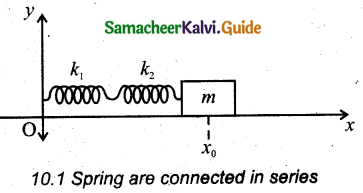Let F be the applied force towards right as shown in Figure. The net displacement of the mass point is
x = x1 + x2
From Hooke’s law, the net force,
F = – ks(x1 + x2)
The effective spring constant can be calculated asWhen n springs connected in series, the effective spring constant in series isIf all spring constants are identical
i.e., k1 = k2 = … = kn = k then
$$\frac{1}{k_{s}}$$ = $$\frac { n }{ k }$$ ⇒ ks = $$\frac { k }{ n }$$
This means that the effective spring constant reduces by the factor n. So, for springs in series connection, the effective spring constant is lesser than the individual spring constants.

Question 7.
Write short notes on two springs connected in parallel.
Let us consider only two springs of spring constants k1 and k2 attached to a mass m as shown in Figure.Net force for the displacement of mass m is F = – kpx … (1)
Where kp is called effective spring constant. Let the first spring be elongated by a displacement x due to force F1 and the second spring be elongated by the same displacement x due to force F2, then the net force
F = – k1x – k2x … (2)
Equating equations (2) and (1), we get
kp = k1 + k2 … (3)
Generalizing, for n springs connected in parallel,
kp = $$\sum_{i=1}^{n} k_{i}$$ … (4)
If all spring constants are identical i.e., k1 = k2 = … = kn = k then
kp = nk … (5)
It is implied that the effective spring constant increases by a factor n. So, for the springs in parallel connection, the effective spring constant is greater than the individual spring constant.

Question 8.
Write down the time period of simple pendulum.
T = 2π$$\sqrt{\frac{l}{g}}$$ in second.
where l – length of the pendulum.
g – acceleration due to gravity.Question 9.
State the laws of simple pendulum.
Laws of simple pendulum: The time period of a simple pendulum.

Depends on the following laws:
(i) Law of length: For a given value of acceleration due to gravity, the time period of a simple pendulum is directly proportional to the square root of length of the pendulum.
T ∝ $$\sqrt{l}$$ … (1)

(ii) Law of acceleration: For a fixed length, the time period of a simple pendulum is inversely proportional to the square root of acceleration due to gravity.
T ∝ $$\frac{1}{\sqrt{g}}$$ … (2)

Question 10.
Write down the equation of time period for linear harmonic oscillator.
Time period T = $$\frac { 1 }{ f }$$ = 2π $$\sqrt{\frac{m}{k}}$$s
where m is the mass, k is the spring constant.

Question 11.
What is meant by free oscillation?
The oscillations in which the amplitude decreases gradually with the passage of time are called damped Oscillations.
Example:

1. The oscillations of a pendulum or pendulum oscillating inside an oil-filled container.
2. Electromagnetic oscillations in a tank circuit.
3. Oscillations in a dead beat and ballistic galvanometers.

Question 12.
Explain damped oscillation. Give an example.
If an oscillator oscillates in a resistive medium, then its amplitude goes on decreasing. The motion of the oscillator is called damped oscillation.
Example:

• The oscillations of a pendulum (including air friction) or pendulum oscillating inside an oil-filled container.
• Electromagnetic oscillations in a tank circuit.

Question 13.
Define forced oscillation. Give an example.
The body executing vibration initially vibrates with its natural frequency and due to the presence of external periodic force, the body later vibrates with the frequency of the applied periodic force. Such vibrations are known as forced vibrations.
Example: Soundboards of stringed instruments.Question 14.
What is meant by maintained oscillation? Give an example.
To avoid damping in an oscillation, energy is supplied from an external source, the amplitude of the oscillation can be made constant. Such vibrations are known as maintained vibrations.

Example:
The vibration of a tuning fork getting energy from a battery or from an external power supply.

Question 15.
Explain resonance. Give an example.
The frequency of external periodic force (or driving force) matches with the natural frequency of the vibrating body (driven). As a result, the oscillating body begins to vibrate such that its amplitude increases at each step and ultimately it has a large amplitude. Such a phenomenon is known as resonance and the corresponding vibrations are known as resonance vibrations. Example: The breaking of glass due to soundQuestion 1.
What is meant by simple harmonic oscillation? Give examples and explain why every simple harmonic motion is a periodic motion whereas the converse need not be true.
Simple harmonic motion is a special type of oscillatory motion in which the acceleration or force on the particle is directly proportional to its displacement from a fixed point and is always directed towards that fixed point.

Example: Oscillation of a pendulum. SHM is a special type of periodic motion, where restoring force is proportional to the displacement and acts in the direction opposite to displacement.

Question 2.
Describe Simple Harmonic Motion as a projection of uniform circular motion.
(i) Let us consider a particle of mass m moving with uniform speed v along the circumference of a circle whose radius is r in an anti-clockwise direction (as shown in Figure).

(ii) It is assumed that the origin of the coordinate System coincides with the center 0 of the circle.

(iii) If co is the angular velocity of the particle and 0 the angular displacement of the particle at any instant of time t, then θ = ωt. By projecting the uniform circular motion on its diameter a simple harmonic motion is obtained.

(iv) This means that we can associate a map (or a relationship) between uniform circular (or revolution) motion to vibratory motion.

(v) Conversely, any vibratory motion or revolution can be mapped to uniform circular motion. The position of a particle moving is projected on to its vertical diameter or on to a line parallel to vertical diameter.

(vi) Similarly, we can do it for horizontal axis or a line parallel to the horizontal axis.

Example: Let us consider a spring-mass system (or oscillation of pendulum) as shown in Figure. When the spring moves up and down (or pendulum moves to and fro), the motion of the mass or bob is mapped to points on the circular motion.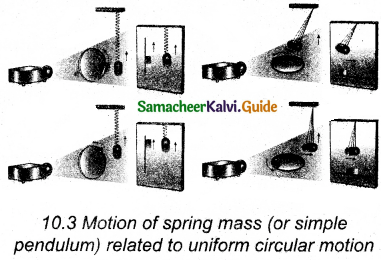Thus, if a particle undergoes uniform circular motion then the projection of the particle on the diameter of the circle (or on a line parallel to the diameter) traces straight line motion that is simple harmonic in nature. The circle is known as the reference circle of the simple harmonic motion.

Question 3.
What is meant by angular harmonic oscillation? Compute the time period of angular harmonic oscillation.If a body is allowed to rotate freely about a given axis then the oscillation is known as the angular oscillation. The point at which the resultant torque acting on the body is taken to be zero. It is called mean position. If the body is displaced from the mean position, then the resultant torque acts such that it is proportional to the angular displacement. This torque has a tendency to bring the body towards the mean position.

Time period : Let $$\overline{θ}$$ be the angular displacement of the body and the resultant torque $$\vec { τ }$$ acting on the body is,
$$\vec { τ }$$ ∝ $$\vec {θ}$$ … (1)
$$\vec { τ }$$ = – k$$\vec {θ}$$ … (2)
K is the restoring torsion constant, that is torque per unit angular displacement. If I is the moment of inertia of the body and $$\vec { τ }$$ ∝ $$\vec {α}$$ is the angular acceleration then
$$\vec { τ }$$ = I $$\vec{α}$$ = k $$\vec {θ}$$
But $$\vec{α}$$ = $$\frac{d^{2} \vec{\theta}}{d t^{2}}$$ and therefore
$$\frac{d^{2} \vec{\theta}}{d t^{2}}$$ = – $$\frac { k }{ I }$$$$\vec {θ}$$ … (3)
This differential equation resembles simple harmonic differential equation.
By comparing equation (3) with simple harmonic motion given we get,
a = $$\frac{d^{2} y}{d t^{2}}$$ = – ω²y, we have
ω = $$\sqrt{\frac{k}{I}}$$ rads-1 … (4)
The frequency of the angular harmonic motion is given equation ω = 2πf is
f = $$\frac { 1 }{ 2π }$$ $$\sqrt{\frac{k}{I}}$$Hz … (5)
The time period is given equation is
T = $$\frac { 1 }{ f }$$; T = 2π$$\sqrt{\frac{I}{k}}$$ second
In angular simple harmonic motion, the displacement of the particle is measured in terms of angular displacement $$\vec {θ}$$.Question 4.
Write down the difference between simple harmonic motion angular simple harmonic motion.
Comparison of simple harmonic motion and angular simple harmonic motion.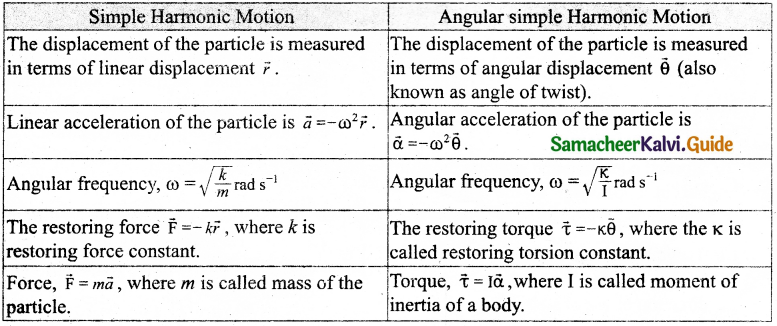Question 5.
Discuss the simple pendulum in detail.
Construction:
A pendulum is a mechanical system which exhibits periodic motion. It has a bob with mass m suspended by a massless and inextensible string. The other end is fixed on a stand as shown in Figure (a). At equilibrium, the pendulum does not oscillate and is suspended vertically downward.

Such a position is known as mean position or equilibrium position. When a pendulum is displaced through a small displacement from its equilibrium position and released, the bob of the pendulum executes to and fro motion.Calculation of time period: Let l be the length of the pendulum which is taken as the distance between the point of suspension and the centre of gravity of the bob. Two forces act on the bob of the pendulum at any displaced position, as shown in Figure (d),

• The gravitational force acting on the body (F = mg) acts vertically downwards.
• The tension in the string T acts along the string to the point of suspension.

Resolving the gravitational force into its components:

• Normal component: It is along the string but in opposition to the direction of tension, Fas = mg cosθ.
• Tangential component: It is perpendicular to the string i.e., along the tangential direction of arc of the swing, Fps = mg sinθ.

Hence, the normal component of the force is, along the string,
T – Was = m$$\frac{v^{2}}{l}$$
Here v is speed of bob
T – mg cosθ = m$$\frac{v^{2}}{l}$$ … (1)
From the Figure, it is observed that the tangential component Wps of the gravitational force always points towards the equilibrium position. This direction always points opposite to the direction of displacement of the bob from the mean position. Hence, in this case, the tangential force is the restoring force. Applying Newton’s second law along tangential direction, we haveWhere, s is the position of bob that is measured along the arc. Expressing arc length in terms of angular displacement i.e.,
s = lθ … (3)
Then its acceleration,
$$\frac{d^{2} \theta}{d t^{2}}$$ = $$\frac{d^{2} \theta}{d t^{2}}$$ … (4)
Substituting equation (4) in equation (2), we get,
l$$\frac{d^{2} \theta}{d t^{2}}$$ = – g sin θ
$$\frac{d^{2} \theta}{d t^{2}}$$ = – $$\frac { g }{ l }$$ sin θ … (5)
Because of the presence of sinθ in the above differential equation, it is a nonlinear differential equation. It is assumed that “the small oscillation approximation”, sin θ ≈ θ, the above differential equation becomes linear differential equation.
$$\frac{d^{2} \theta}{d t^{2}}$$ = – $$\frac { g }{ l }$$ θ
It is known as oscillatory differential equation. Hence, the angular frequency of this oscillator (natural frequency of this system) isQuestion 6.
Explain the horizontal oscillations of a spring.Let us consider a system containing a block of mass m fastened to a massless spring with stiffness constant or force constant or spring constant k placed on a smooth horizontal surface (frictionless surface) as shown in Figure. Let x0 be the equilibrium position or mean position of mass m when it is left undisturbed.

When the mass is displaced through a small displacement x towards right from its equilibrium position and then released, it will oscillate back and forth about its mean position x0. Let F be the restoring force (due to stretching of the spring) that is proportional to the amount of displacement of block: For one-dimensional motion, we get
F ∝ x
F = – kx
where negative sign implies that the restoring force will always act opposite to the direction of the displacement. This equation is called Hooke’s law. It is noticed that, the restoring force is linear with the displacement (i.e., the exponent of force and displacement are unity). This is not always true. If we apply a very large stretching force, then the amplitude of oscillations becomes very large.
m$$\frac{d^{2} x}{d t^{2}}$$ = – kx
$$\frac{d^{2} x}{d t^{2}}$$ = – $$\frac { k }{ m }$$x … (1)
Comparing the equation (1) with simple harmonic motion equation a = $$\frac{d^{2} y}{d t^{2}}$$ = – ω²y, we get
ω² = $$\frac { k }{ m }$$
which means the angular frequency or natural frequency of the oscillator is
ω = $$\sqrt{\frac{k}{m}}$$rad s-1 … (2)
The frequency of the oscillation is
f = $$\frac { ω }{ 2π }$$ = $$\frac { 1 }{ 2π }$$$$\frac { k }{ m }$$ Hertz … (3)
and the time period of the oscillation is
T = $$\frac { 1 }{ f }$$ = 2π[/latex]$$\frac { m }{ k }$$ seconds … (4)

Question 7.
Describe the vertical oscillations of a spring.
Consider a massless spring with stiffness constant or force constant k attached to a ceiling as shown in Figure. Let the length of the spring before loading mass m be L. If the block of mass m is attached to the other end of the spring, then the spring elongates by a length l. Let F1 be the restoring force due to the stretching of spring. Due to mass m, the gravitational force acts vertically downward. A free-body diagram is drawn for this system as shown in Figure. When the system is under equilibrium,F1 + mg = 0 … (1)
But the spring elongates by small displacement l,
∴ F1 ∝ l ⇒ F1 = – kl … (2)
Substituting equation (2) in equation (1), we get
– kl + mg = 0
mg = kl
(or) $$\frac { m }{ k }$$ = $$\frac { 1 }{ g }$$ … (3)
Suppose a very small external force is applied on the mass such that the mass further displaces downward by a displacement y, then it will oscillate up and down. Now, the restoring force due to this stretching of spring (total extension of spring is y + l) is
F2 (y + 1)
F2 = – k(y + 1) = – ky – kl … (4)
Since, the mass moves up and down with acceleration , by drawing the free body diagram for this case, we get
-ky – kl + mg = m$$\frac{d^{2} y}{d t^{2}}$$ … (5)
The net force acting on the mass due to this stretching is
F = F2 + mg
F = – ky – kl + mg … (6)
The gravitational force opposes the restoring force. Substituting equation (3) in equation (6), we get
F = – ky – kl + kl = ky
Time period:
Applying Newton’s law, we get
m$$\frac{d^{2} y}{d t^{2}}$$ = – ky
$$\frac{d^{2} y}{d t^{2}}$$ = – $$\frac { k }{ m }$$y … (7)
The above equation is in the form of simple harmonic differential equation. Hence the time period is
T = 2π $$\sqrt{\frac{m}{k}}$$second … (8)
The time period can be rewritten using equation (3) as
T = 2π $$\sqrt{\frac{m}{k}}$$ = 2π $$\sqrt{\frac{l}{g}}$$second.Question 8.
Write short notes on the oscillations of the liquid column in U-tube.
Let us consider a U-shaped glass tube which consists of two open arms with uniform cross¬sectional area A. Let us pour a non-viscous uniform incompressible liquid of density p in the U-shaped tube to a height h as shown in the Figure. If the liquid and tube are not disturbed then the liquid surface will be in equilibrium position O.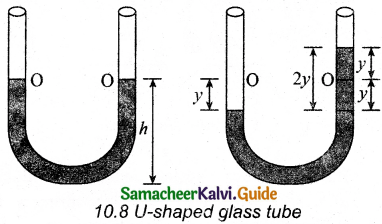It means the pressure as measured at any point on the liquid is the same and also at the surface on the arm (edge of the tube on either side), that balances the atmospheric pressure. Hence, the level of liquid in each arm will be the same. By blowing air one can provide sufficient force in one arm, and the liquid gets disturbed from equilibrium position O, It is meant, that pressure at blown arm is higher than the other arm.

A difference in pressure is created that will cause the liquid to oscillate for a very short duration of time about the mean or equilibrium position. Finally, it comes to rest.
Time period of the oscillation is
T= 2π $$\sqrt{\frac{l}{2g}}$$second

Question 9.
Discuss in detail the energy in simple harmonic motion.
(i) Expression for Potential Energy:For the simple harmonic motion, the force and the displacement are related by Hooke’s law
$$\vec { F }$$ = – k$$\vec { r }$$
F = – kx … (1)
the work done by the conservative force field is independent of path.
Calculation potential energy:
F = $$\frac { dU }{ dx }$$ … (2)
Comparing (1) and (2), we get
$$\frac { dU }{ dx }$$ = – kx
dU = – kx dx
This work done by the force F during a small displacement dx stores as potential energyFrom equation,
ω = $$\sqrt{\frac{k}{m}}$$ rad s-1
By substituting the value of force constant k = mω² in equation (3), we get
U(x) = $$\frac { 1 }{ 2 }$$mω²x² … (4)
where ω is the natural frequency of the oscillating system. For the particle executing simple harmonic motion from the equation,
y = A sin ωt
we get,
x = A sin ωt
U(t) = $$\frac { 1 }{ 2 }$$mω²x² … (5)
This variation of U is shown below.(ii) Expression for Kinetic Energy:
Kinetic energy
KE = $$\frac { 1 }{ 2 }$$mvx² = $$\frac { 1 }{ 2 }$$m($$\frac { dx }{ dt }$$)² … (6)
Since the particle executes simple harmonic motion, from equation y = A sin ωt
x = A sin ωt
∴ velocity is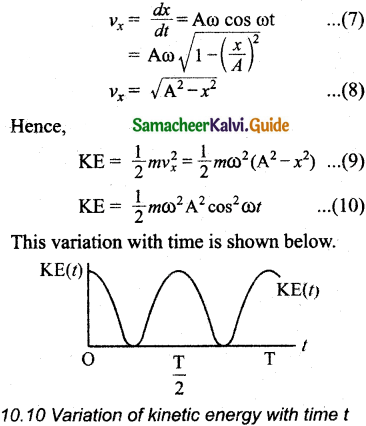(iii) Expression for Total Energy: Total energy is the sum of kinetic energy and potential energy.
E = KE + U … (11)
E = $$\frac { 1 }{ 2 }$$mω²(A² – x²) + $$\frac { 1 }{ 2 }$$mω²x² … (12)
Hence, cancelling x² term,
E = $$\frac { 1 }{ 2 }$$mω²x² = r constant … (13)
Alternatively, from equation (5) and equation (10), we get the total energy as
E = $$\frac { 1 }{ 2 }$$mω²x² sin²ωt + $$\frac { 1 }{ 2 }$$mω²A²cos²ωt
= $$\frac { 1 }{ 2 }$$mω²A² (sin²ωt + cos²ωt)
From trigonometry identity,
(sin² ωt + cos² ωt) = 1
E = $$\frac { 1 }{ 2 }$$mω²A² = constant
which gives the law of conservation of total energy.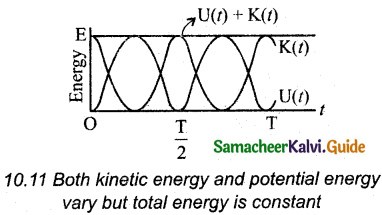Question 10.
Explain in detail the four different types of oscillations.
(i) Free oscillations: When the oscillator oscillates with a frequency that is equal to the natural frequency of the oscillator. Such an oscillation or vibration is known as free oscillation or free vibration.

Example:

• Oscillation of a simple pendulum,
• Vibration in a stretched string.

(ii) Damped oscillation: If an oscillator oscillates in a resistive medium, then its amplitude goes on decreasing. The energy of the oscillator is used to do work against the resistive medium. The motion of the oscillator is said to be a damped oscillation.

Example:

• The oscillations of a pendulum (including air friction) or pendulum oscillating inside an oil filled container.
• Electromagnetic oscillations in a tank circuit.

(iii) Forced oscillations: In this type of vibration, the body executing vibration initially vibrates with its natural frequency. Because of the presence of external periodic force, the body later vibrates with the frequency of the applied periodic force. Such vibrations are known as forced vibrations.

Example:
Soundboards of stringed instruments.

(iv) Maintained oscillations: The amplitude of the oscillation can be made constant. By supplying energy from an external source. Such oscillations are known as maintained oscillations.

Example:
The vibration of a tuning fork getting energy from a battery or from external power supply.IV. Numerical Problems:

Question 1.
Consider the Earth as a homogeneous sphere of radius R and a straight hole is bored in it through its centre. Show that a particle dropped into the hole will execute a simple harmonic motion such that its time Period is T = 2π$$\sqrt{\frac{\mathrm{R}}{g}}$$(Here, negative has no meaning. It can be neglected)
Comparing equation (1) & (2) we get,Time period, T = 2π$$\sqrt{\frac{\mathrm{R}}{g}}$$
Hence proved.

Question 2.
Calculate the time period of the oscillation of a particle of mass m moving in the potential defined as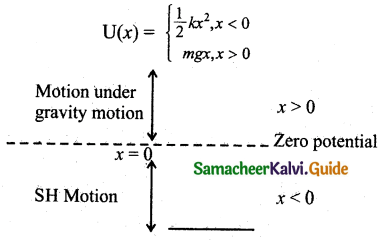In the problem consider oscillation of particle into 2 cases via x < 0 (consider it as SHM where the time period is considered as t1) and another one as x > 0 (consider it as a motion under gravity, where time period is t2)

Note:
We want to find the total time period, which will be T = t1 + t2.
According to the conservation of energy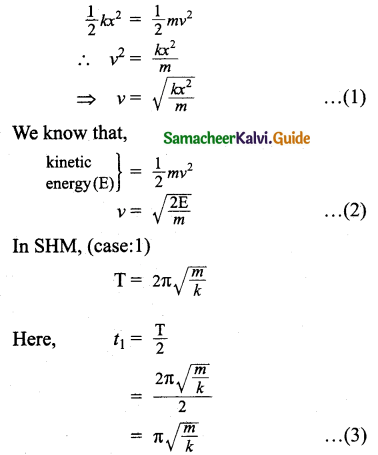In case: 2 (Motion under gravity)
t2 = $$\frac { 2v }{ g }$$ substituting equation (2) here we get,
t2 = $$\frac { 2 }{ g }$$$$\sqrt{\frac{2 E}{m}}$$
⇒ 2$$\sqrt{\frac{2 \mathrm{E}}{m g^{2}}}$$ … (4)
Time period of oscillation,
T = t1 + t2
= π$$\sqrt{\frac{m}{k}}$$ + 2$$\sqrt{\frac{2 \mathrm{E}}{m g^{2}}}$$

Question 3.
Consider a simple pendulum of length l = 0.9 m which is properly placed on a trolley rolling down on a inclined plane which is at θ = 45° with the horizontal. Assuming that the inclined plane is frictionless, calculate the time period of oscillation of the simple pendulum.
The effective value of acceleration due to gravity (g) will be equal to the component of g normal to the inclined plane which is
g’ = g cos α
T = 2π$$\sqrt{\frac{l}{g^{\prime}}}$$ = 2π$$\sqrt{\frac{l}{g \cos \theta}}$$
Length of the pendulum l = 0.9m
Angle of inclination θ = 45°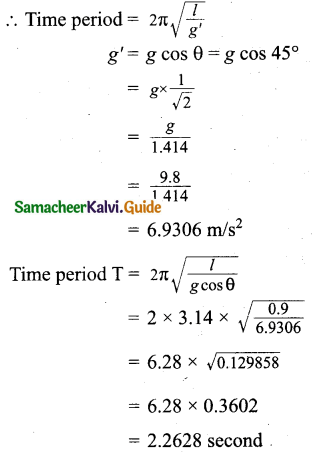Question 4.
A piece of wood of mass m is floating erect in a liquid whose density is ρ. If it is slightly pressed down and released, then executes simple harmonic motion. Show that its time period of oscillation is T = 2π$$\sqrt{\frac{m}{\mathrm{Ag} \rho}}$$
When a wood is pressed and released,Question 5.
Consider two simple harmonic motion along x and y-axis having same frequencies but different amplitudes as x = A sin (ωt + φ) (along x axis) and y = B sin ωt (along y axis). Then show that $$\frac{x^{2}}{\mathrm{~A}^{2}}+\frac{y^{2}}{\mathrm{~B}^{2}}-\frac{2 x y}{\mathrm{AB}}$$ cosφ = sin²φ and also discuss the special cases when
(i) φ = 0
(ii) φ = π
(iii) φ = $$\frac { π }{ 2 }$$
(iv) φ = $$\frac { π }{ 2 }$$ and A = B
(v) φ = $$\frac { π }{ 4 }$$
Note: when a particle is subjected to two simple harmonic motions at right angle to each other the particle may move along different paths. Such paths are called Lissajous figures.
Given:
x = A sin(ωt-φ) … (1)
y = B sin ωt … (2)
In equation (1) use,
sin (A – B) – sin A cos B + cos A sin B
(1) ⇒ x – A sin ωt. cos (φ) + A cos ωt. sinφ
x – A sin cat. cos φ = A cos cot sin φ
squaring on both sides we get,
(x – A sin cot. cos φ)² = A² cos²cot sin²φ … (3)
In equation (3) sin at can be re-written as, $$\frac { y }{ B }$$ [from equation (2)]. Also, use
cos²cot = 1 – sin²ωt in equation (3)
∴ (3) becomes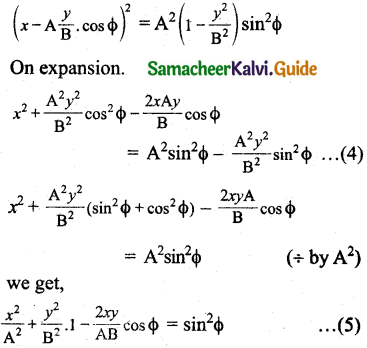Hence proved.

Special cases:
(i) φ = 0 in equation (5) we get,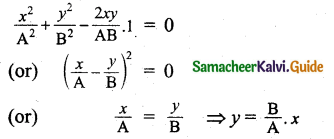The above equation resembles equation of a straight line passing through origin with positive slope.

(ii) φ = π in equation (5)The above equation is an equation of a straight line passing through origin with a negative slope.

(iii) φ = $$\frac { π }{ 2 }$$ in equation (5)
The above equation of an ellipse whose centre is origin.

(iv) φ = $$\frac { π }{ B }$$ and A = B in equation (5)
$$\frac{x^{2}}{\mathrm{~A}^{2}}+\frac{y^{2}}{\mathrm{~A}^{2}}$$ = 1
⇒ x² + y² = A²
The above equation of a circle whose centre is origin.

(v) φ = $$\frac { π }{ 4 }$$, cos $$\frac { π }{ 4 }$$ = $$\frac{1}{\sqrt{2}}$$ sin$$\frac { π }{ 4 }$$ = $$\frac{1}{\sqrt{2}}$$ equation (5) we get,
$$\frac{x^{2}}{\mathrm{~A}^{2}}+\frac{y^{2}}{\mathrm{~A}^{2}}$$ – $$\frac{(\sqrt{2}) x y}{A B}$$ = $$\frac { 1 }{ 2 }$$
The above equation is an equation of tilted ellipse.Question 6.
Show that for a particle executing simple harmonic motion,
(a) the average value of kinetic energy is equal to the average value of potential energy,
(b) average potential energy = average kinetic energy = $$\frac { 1 }{ 2 }$$ (total energy)
Hint: average kinetic energy = < kinetic energy > = $$\frac{1}{\mathrm{~T}} \int_{0}^{\mathrm{T}}(\text { Potential energy }) d t$$ and average potential energy = < potential energy > = $$\frac{1}{\mathrm{~T}} \int_{0}^{\mathrm{T}}(\text { Potential energy }) d t$$
(a) Suppose a particle of mass m executes SHM of time period T. The displacement of the particle at any instant t is given by
y = A sin ωr … (1)
Velocity v = $$\frac { dy }{ dt }$$ = $$\frac { d }{ dt }$$(sin ωt) = Aω cos ωt = ωA cosωt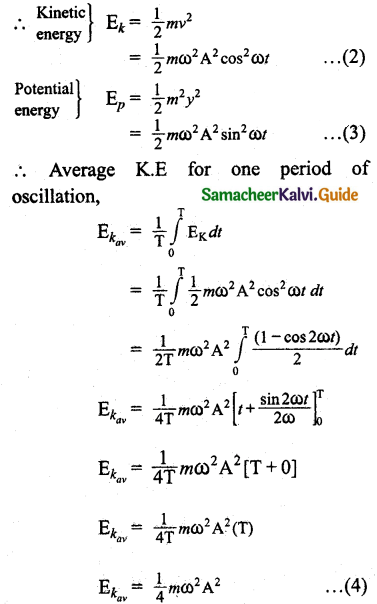Average potential energy over a period of oscillation is,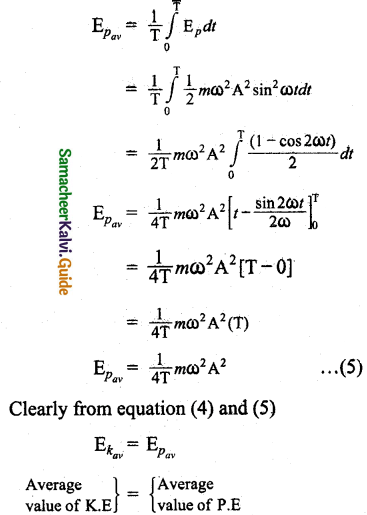(b) Total energy
T.E = $$\frac { 1 }{ 2 }$$ ω²y² + $$\frac { 1 }{ 2 }$$mω²(A² – y²)
But y = A sinωt
T.E = $$\frac { 1 }{ 2 }$$mω²A² ωt + $$\frac { 1 }{ 2 }$$mω²A² cos²ωt
= $$\frac { 1 }{ 2 }$$mω²A²(sin²ωt + cos²ωt)
From trignometry identity
sin² ωt + cos² ωt = 1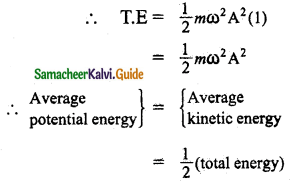Question 7.
Compute the time period for the following system if the block of mass m is slightly displaced vertically down from its equilibrium position and then released. Assume that the pulley is light and smooth, strings and springs are light.Time period T = $$\sqrt{\frac{m}{k}}$$
∴ T = 2π$$\sqrt{\frac{m}{4k}}$$